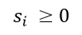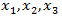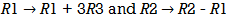Goseeko blog# What is simplex method?

The simplex method is one of the most powerful and popular linear programming methods. The simplex method is an iterative procedure to get the most viable solution. This method keeps transforming the values   of the fundamental variables to get the maximum value of the objective function.

Linear programming functions are in standard form when trying to maximize the objective function. Subject to constraints,

Here and after adding the Slack variable, the system of corresponding constraint equations Where,S1, s2…………sm is called a slack variable. These are non-negative numbers that are added to remove the inequality from the equation.

## Solved example-1

Example: Maximize-

Subject to-

By using simplex method.

Solution

Here we have

And  the inequalities-

By adding s1 and s2 we get-

Putting decision variablesequals to zero, we get-

Since the coefficient (–3) of  x3 is most negative, so x3 is an entering variable.

The smallest ratio is 1 in the s2-row, therefore s2 is the departing variable. The pivot entry (2) is at the intersection of x3-column and s2-row.

To make pivot entry (1), we multiply third row by ½, we get-

Applying, we get

Since, Z row of the table has non-negative entries in the column of variables, therefore, this is the case of optimal solution. From the last column of the table we have x1 = 0, x2 = 0 and x3 = 1 and the maximum value of Z = 3.

## Solved example-2

Solve a product-mix problem for which the values of ?1 and ?2 that

Maximize: ? = 3?1 +2?2

Subject to: −?1 + 2?2 ≤ 4

3?1 + 2?2 ≤ 14

?1 − ?2 ≤ 3

?1 ≥ 0 , ?2 ≥ 0

Sol:

The solution process to maximize first introduce the slack variables ?1 , ?2 ??? ?3 . Hence

????????: ? = 3?1 + 2?2 +0. ?1 +0. ?2 + 0. ?3

We get

?1 + 2?2 +?1 +0. ?2 + 0. ?3 = 4

3?1 +2?2 + 0. ?1 +?2 +0. ?3 = 14

?1 − ?2 + 0. ?1 + 0. ?2 + ?3 = 3

?1 , ?2 , ?1 , ?2 , ?3 ≥ 0

From the table the pivot element is 1 hence the pivot column is the first column and the pivot row is the third row because of the most negative number on that column and least positive ratio respectively.

Here again the second column is the pivot column and the second row is also pivot row.

If the value of ?? − (contribution of unit price to the profit) are all positive or zero, the current basic feasible solution is optimal. But there values one or more negative choose the variable corresponding to which the value of ?? − ?? is least i.e most negative.

Hence the optimal solution will be-

And Max. Z = 14

Interested in learning about similar topics? Here are a few hand-picked blogs for you!